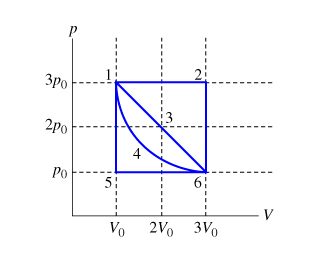# Problem: Calculate the work W done by the gas during process 1→2. Express your answer in terms of p0 and V0.

###### FREE Expert Solution

Work done in a constant pressure process is given by:

$\overline{){\mathbf{W}}{\mathbf{=}}{\mathbf{P}}{\mathbf{·}}{\mathbf{∆}}{\mathbf{V}}}$

P = 3P0

90% (130 ratings)###### Problem Details

Calculate the work W done by the gas during process 1→2. Express your answer in terms of p0 and V0.